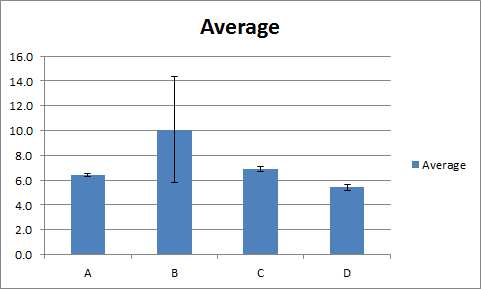### Replicates and independent experiments

Continuing from my last post about accuracy and precision, I would like to write about replicate experiments and independent experiments.

Replicate experiments are the ones you do simultaneously. For example, you set up 3 reactions in three separate test tubes to detect glucose concentration from a single sample. These are replicate experiments. Results of replicate experiments reflect your working hand (like pippeting errors). If results from all replicates are close enough or are same, then you can only say that you did the assay very nicely. However it does not tell you that (in the example above) the glucose concentration in the material from which you extracted glucose (test material) is same as what you have got from the assay results. For you to say this, you need to do independent experiments.

Again continuing with the example, you need to grow the organism (or essentially repeat the whole experiment) once again and do the assays.  It is helpful to do replicate experiments within each of the independent experiments you do, again, to monitor your working hand. If you get the assay results similar to what you got in the previous experiment then you can that the glucose concentration in the test material is close to the value you got from your assays.

Results of replicate experiments reflect the precision with which you did your experiments while the results of independent experiments reflect how accurate your results are.

I am showing an example data below:

 Glucose concentration (mg/g) EX1 EX2 EX3 Average S.D. A 6.3 6.5 6.4 6.4 0.1 B 7.7 15 7.5 10.1 4.3 C 6.9 6.7 7.1 6.9 0.2 D 5.3 5.7 5.3 5.4 0.2

The data shows glucose concentrations per gram of test materials, A,B,C,and D. Each of the values under columns EX1,EX2 and EX3 are averages of three values got from replicate experiments (We will assume that these values were quite close to each other).  All the results of replicate experiments should make up only one data point.
Last two columns show the average values and standard deviations of the separate data points from three independent experiments.
While plotting this data as 'clustered columns' in excel, we should use the average values as height (y-axis) of the columns and standard deviation values as the error bars.We can clearly see that in samples A,B and D, the error bars are very small. This means that the glucose concentrations obtained from our experiments are fairly accurate. However, the standard deviation is too high in the sample B. No matter how close the obtained values were among replicate experiments, the results of independent experiments had quite a lot difference. This simply means that the experiments were performed with precision, but the accuracy of the results are questionable.

While plotting data, one should not add error bars for results of replicate experiments. They all form only one data point. In this example each experiment was done with three replicates, average of which formed one data point. Three such experiments formed three data points (n=3) average of which was plotted in the graph  with error bars representing the standard deviation.